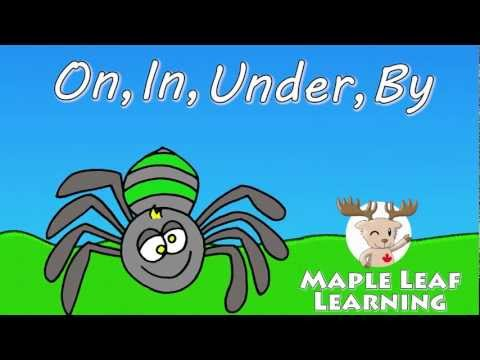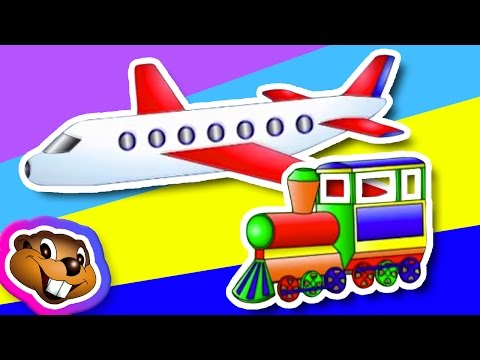# 8. in/on/under/by

3. klass > Inglise keel > Õppeaasta

###Kus on ämblik? Where is the spider?### Laul: on, in, under, in front of, behind, next to, between Kus on hiir? (Where is the mouse?) Hiir on majas. (The mouse is in the house.) Kus on kass? (Where is the cat?) Kass on mütsi sees. (The cat is in the hat.) Kus on kala? (Where is the fish?) Kala on nõus. (The fish is in the dish.) Kus sina oled? (Where are you?) Ma olen klassis. (I’m in the classroom.) Ees – in front of Taga – behind Kõrval – next to Vahel – between Kus on karu? (Where is the bear?) Karu on toolil. (The bear is on the chair.) Kus on madu. (Where is the snake?) Madu on koogil. (The snake is on the cake.) Kus on kitarr? (Where is the guitar?) Kitarr on auto peal. (The guitar is on the car.) Kus oled sina? (Where are you?) Ma olen ka toolil. (I’m on the chair too.) Kus on rebane? (Where is the fox?) Rebane on kasti all. (The fox is under the box.) Kus on rong? (Where is the train?) Rong on lennuki all. (The train is under the airplane.) Kus on roos? (Where is the rose?) Roos on nina all. (The rose is under the nose.) Kus teie olete? (Where are you?) Meie oleme kuu all. (We’re under the moon.) Kus on D? (Where is the D?) D on C ees. (The D is in front of the C.) Kus on C? (Where is the C?) C on D taga. (The C is behind the D.) Kus on E? (Where is the E?) E on D kõrval. (The E is next to the D.) Kus oled sina? (Where are you?) Ma olen M ja W vahel. (I’m between M and W.)